Group based trajectory models in Stata – some graphs and fit statistics

For my advanced research design course this semester I have been providing code snippets in Stata and R. This is the first time I’ve really sat down and programmed extensively in Stata, and this is a followup to produce some of the same plots and model fit statistics for group based trajectory statistics as this post in R. The code and the simulated data I made to reproduce this analysis can be downloaded here.

First, for my own notes my version of Stata is on a server here at UT Dallas. So I cannot simply go

net from http://www.andrew.cmu.edu/user/bjones/traj
net install traj, force

to install the group based trajectory code. First I have this set of code in the header of all my do files now

*let stata know to search for a new location for stata plug ins
*to install on your own in the lab it would be

So after running that then I can install the traj command, and Stata will know where to look for it!

Once that is taken care of after setting the working directory, I can simply load in the csv file. Here my first variable was read in as Ã¯id instead of id (I’m thinking because of the encoding in the csv file). So I rename that variable to id.

import delimited GroupTraj_Sim.csv
*BOM mark makes the id variable weird
rename Ã¯id id

Second, the traj model expects the data in wide format (which this data set already is), and has counts in count_1, count_2count_10. The traj command also wants you to input a time variable though to, which I do not have in this file. So I create a set of t_ variables to mimic the counts, going from 1 to 10.

*Need to generate a set of time variables to pass to traj, just label 1 to 10
forval i = 1/10 {
generate t_`i' = `i'
}

Now we can estimate our group based models, and we get a pretty nice default plot.

traj, var(count_*) indep(t_*) model(zip) order(2 2 2) iorder(0)
trajplot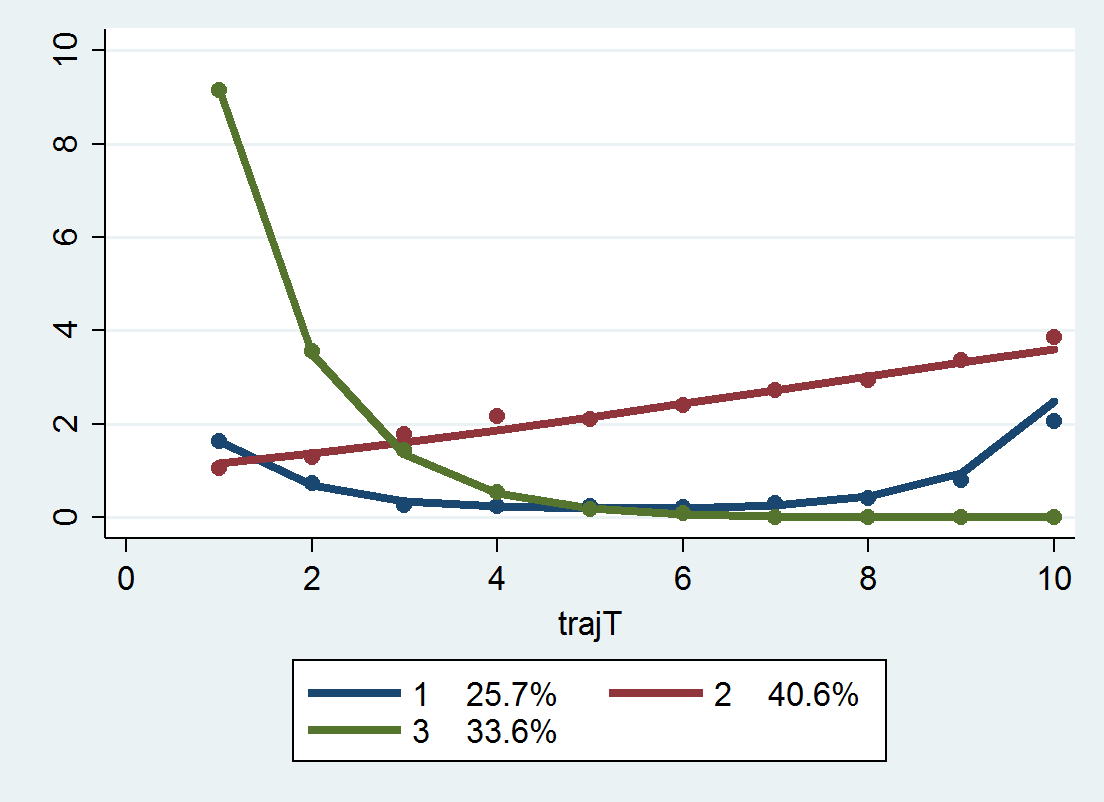Now for absolute model fit statistics, there are the average posterior probabilities, the odds of correct classification, and the observed classification proportion versus the expected classification proportion. Here I made a program that I will surely be ashamed of later (I should not brutalize the data and do all the calculations in matrix), but it works and produces an ugly table to get us these stats.

*I made a function to print out summary stats
program summary_table_procTraj
preserve
*now lets look at the average posterior probability
gen Mp = 0
foreach i of varlist _traj_ProbG* {
replace Mp = `i' if `i' > Mp
}
sort _traj_Group
*and the odds of correct classification
by _traj_Group: gen countG = _N
by _traj_Group: egen groupAPP = mean(Mp)
by _traj_Group: gen counter = _n
gen n = groupAPP/(1 - groupAPP)
gen p = countG/ _N
gen d = p/(1-p)
gen occ = n/d
*Estimated proportion for each group
scalar c = 0
gen TotProb = 0
foreach i of varlist _traj_ProbG* {
scalar c = c + 1
quietly summarize `i'
replace TotProb = r(sum)/ _N if _traj_Group == c
}
gen d_pp = TotProb/(1 - TotProb)
gen occ_pp = n/d_pp
*This displays the group number [_traj_~p],
*the count per group (based on the max post prob), [countG]
*the average posterior probability for each group, [groupAPP]
*the odds of correct classification (based on the max post prob group assignment), [occ]
*the odds of correct classification (based on the weighted post. prob), [occ_pp]
*and the observed probability of groups versus the probability [p]
*based on the posterior probabilities [TotProb]
list _traj_Group countG groupAPP occ occ_pp p TotProb if counter == 1
restore
end

This should work after any model as long as the naming conventions for the assigned groups are _traj_Group and the posterior probabilities are in the variables _traj_ProbG*. So when you run

summary_table_procTraj

You get this ugly table:

| _traj_~p   countG   groupAPP        occ     occ_pp       p    TotProb |
|-----------------------------------------------------------------------|
1. |        1      103   .9379318   43.57342   43.60432   .2575   .2573645 |
104. |        2      136   .9607258   47.48513   48.30997     .34   .3361462 |
240. |        3      161   .9935605   229.0413   225.2792   .4025   .4064893 |

The groupAPP are the average posterior probabilities – here you can see they are all quite high. occ is the odds of correct classification, and again they are all quite high. Update: Jeff Ward stated that I should be using the weighted posterior proportions for the OCC calculation, not the proportions based on the max. post. probability (that I should be using TotProb in the above table instead of p). So I have updated to include an additional column, occ_pp based on that suggestion. I will leave occ in though just to keep a paper trail of my mistake.

p is the proportion in each group based on the assignments for the maximum posterior probability, and the TotProb are the expected number based on the sums of the posterior probabilities. TotProb should be the same as in the Group Membership part at the bottom of the traj model. They are close (to 5 decimals), but not exactly the same (and I do not know why that is the case).

Next, to generate a plot of the individual trajectories, I want to reshape the data to long format. I use preserve in case I want to go back to wide format later and estimate more models. If you need to look to see how the reshape command works, type help reshape at the Stata prompt. (Ditto for help on all Stata commands.)

preserve
reshape long count_ t_, i(id)

To get the behavior I want in the plot, I use a scatter plot but have them connected via c(L). Then I create small multiples for each trajectory group using the by() option. Before that I slightly jitter the count data, so the lines are not always perfectly overlapped. I make the lines thin and grey — I would also use transparency but Stata graphs do not support this.

gen count_jit = count_ + ( 0.2*runiform()-0.1 )
graph twoway scatter count_jit t_, c(L) by(_traj_Group) msize(tiny) mcolor(gray) lwidth(vthin) lcolor(gray)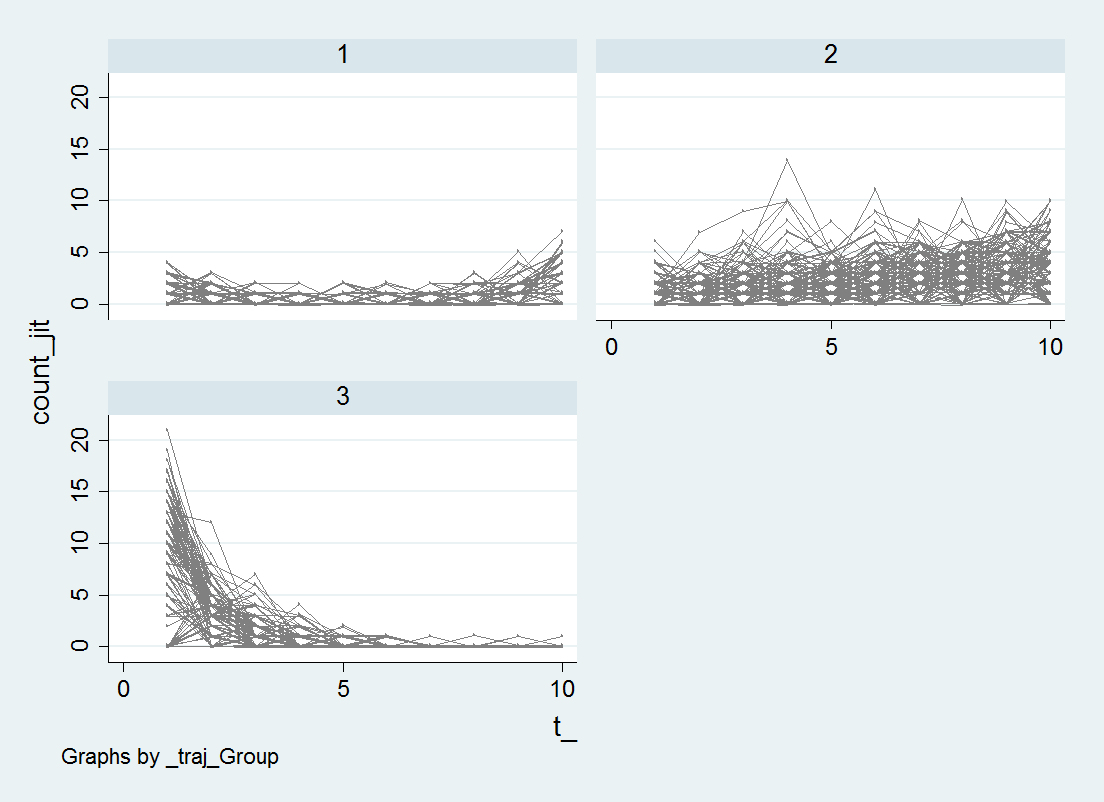I’m too lazy at the moment to clean up the axis titles and such, but I think this plot of the individual trajectories should always be done. See Breaking Bad: Two Decades of Life-Course Data Analysis in Criminology, Developmental Psychology, and Beyond (Erosheva et al., 2014).

While this fit looks good, this is not the correct number of groups given how I simulated the data. I will give those trying to find the right answer a few hints; none of the groups have a higher polynomial than 2, and there is a constant zero inflation for the entire sample, so iorder(0) will be the correct specification for the zero inflation part. If you take a stab at it let me know, I will fill you in on how I generated the simulation.

Plotting panel data with many lines in SPSS

A quick blog post – so you all are not continually assaulted by my mug shot on the front page of the blog!

Panel data is complicated. When conducting univariate time series analysis, pretty much everyone plots the series. I presume people do not do this often for panel data because the charts tend to be more messy and less informative. But by using transparency and small multiple plots are easy places to start to unpack the information. Here I am going to show these using plots of arrest rates from 1970 through 2014 in New York state counties. The data and code can be downloaded here, and that zip file contains info. on where the original data came from. It is all publicly available – but mashing up the historical census data for the population counts by county is a bit of a pain.

So I will start with grabbing my created dataset, and then making a default plot of all the lines. Y axis is the arrest rate per 1,000 population, and the X axis are years.

*Grab the dataset.
FILE HANDLE data /NAME = "!!Your File Handle Here!!!".
GET FILE = "data\Arrest_WPop.sav".
DATASET NAME ArrestRates.

*Small multiple lines over time - default plot.
GGRAPH
/GRAPHDATASET NAME="graphdataset" VARIABLES=Year Total_Rate County
/GRAPHSPEC SOURCE=INLINE.
BEGIN GPL
SOURCE: s=userSource(id("graphdataset"))
DATA: Year=col(source(s), name("Year"))
DATA: Total_Rate=col(source(s), name("Total_Rate"))
DATA: County=col(source(s), name("County"), unit.category())
GUIDE: axis(dim(1), label("Year"))
GUIDE: axis(dim(2), label("Total Arrest Rate per 1,000"))
ELEMENT: line(position(Year*Total_Rate), split(County))
END GPL.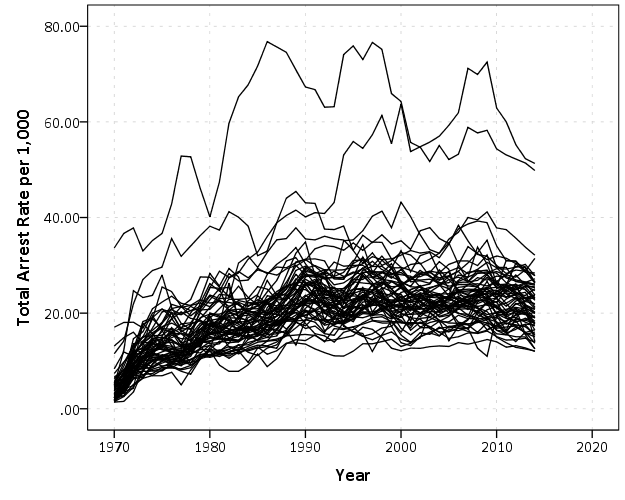That is not too bad, but we can do slightly better by making the lines small and semi-transparent (which is the same advice for dense scatterplots):

*Make them transparent and smaller.
FORMATS Total_Rate (F2.0).
GGRAPH
/GRAPHDATASET NAME="graphdataset" VARIABLES=Year Total_Rate County
/GRAPHSPEC SOURCE=INLINE.
BEGIN GPL
SOURCE: s=userSource(id("graphdataset"))
DATA: Year=col(source(s), name("Year"))
DATA: Total_Rate=col(source(s), name("Total_Rate"))
DATA: County=col(source(s), name("County"), unit.category())
GUIDE: axis(dim(1), label("Year"))
GUIDE: axis(dim(2), label("Total Arrest Rate per 1,000"))
SCALE: linear(dim(1), min(1970), max(2014))
ELEMENT: line(position(Year*Total_Rate), split(County), transparency(transparency."0.7"), size(size."0.7"))
END GPL.This helps disentangle the many lines bunched up. There appear to be two outliers, and basically the rest of the pack.

A quick way to check out each individual line is then to make small multiples. Here I wrap the panels, and make the plot size bigger. I also make the X and Y axis null. This is ok though, as I am just focusing on the shape of the trend, not the absolute level.

*Small multiples.
GGRAPH
/GRAPHDATASET NAME="graphdataset" VARIABLES=Year Total_Rate County
/GRAPHSPEC SOURCE=INLINE.
BEGIN GPL
PAGE: begin(scale(1000px,1000px))
SOURCE: s=userSource(id("graphdataset"))
DATA: Year=col(source(s), name("Year"))
DATA: Total_Rate=col(source(s), name("Total_Rate"))
DATA: County=col(source(s), name("County"), unit.category())
COORD: rect(dim(1,2), wrap())
GUIDE: axis(dim(1), null())
GUIDE: axis(dim(2), null())
GUIDE: axis(dim(3), opposite())
SCALE: linear(dim(1), min(1970), max(2014))
ELEMENT: line(position(Year*Total_Rate*County))
PAGE: end()
END GPL.
*Manually edited to make less space between panels.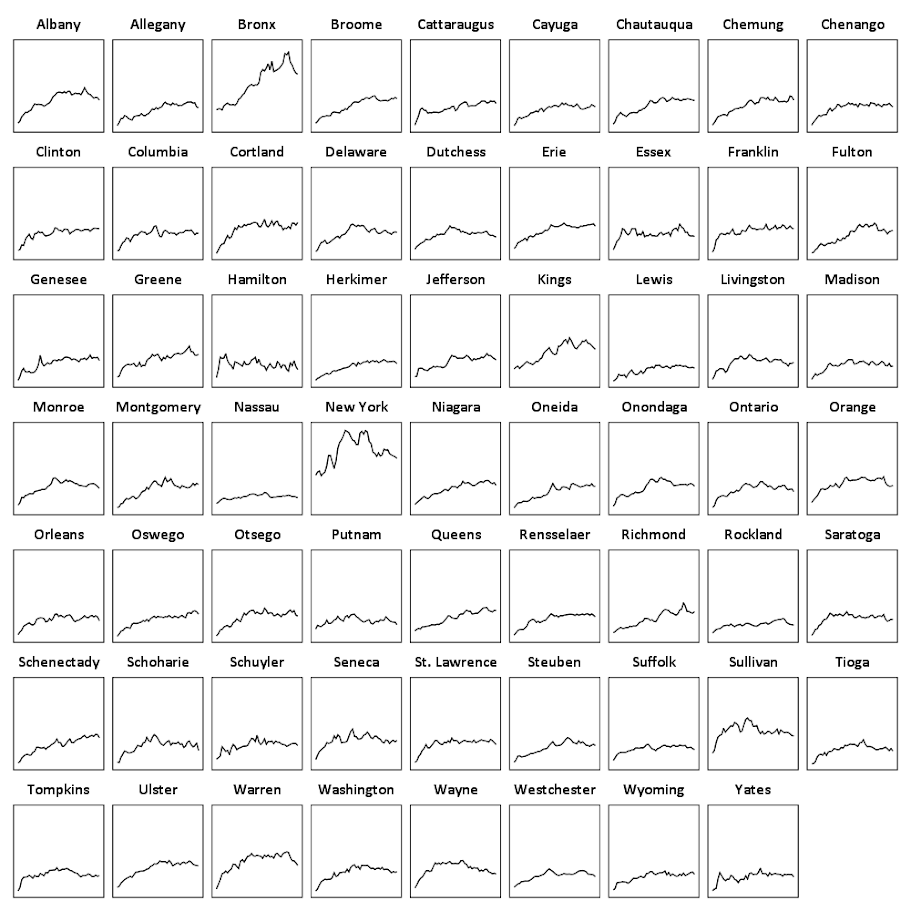There are a total of 62 counties in New York, so this is feasible. With panel sets of many more lines, you can either split the small multiple into more graphs, or cluster the lines based on the overall shape of the trend into different panels.

Here you can see that the outliers are New York county (Manhattan) and Bronx county. Bronx is a pretty straight upward trend (which mirrors many other counties), but Manhattan’s trajectory is pretty unique and has a higher variance than most other places in the state. Also you can see Sullivan county has quite a high rate compared to most other upstate counties (upstate is New York talk for everything not in New York City). But it leveled off fairly early in the time series.

This dataset also has arrest rates broken down by different categories; felony (drug, violent, dwi, other), and misdemeanor (drug, dwi, property, other). It is interesting to see that arrest rates have been increasing in most places over this long time period, even though crime rates have been going down since the 1990’s. They all appear to be pretty correlated, but let me know if you use this dataset to do some more digging. (It appears index crime totals can be found going back to 1990 here.)

Some Stata notes – Difference-in-Difference models and postestimation commands

Many of my colleagues use Stata (note it is not STATA), and I particularly like it for various panel data models. Also one of my favorite parts of Stata code that are sometimes tedious to replicate in other stat. software are the various post-estimation commands. These includes the test command, which does particular coefficient restriction tests or multiple coefficient tests, margins (and the corresponding marginsplot) which gives model based estimates at various values of the explanatory variables, and lincom and nlcom which I will show as being useful for differences in differences models.

To follow along with this post, I have posted all of the data and code here. Part of the data is simulated to be very similar to a panel data model I estimated recently, and the other dataset comes from Dan Kahan.

I don’t know Stata as well as I do SPSS or R, but these are things I tend to re-visit (and wish people sending me data and code follow as well). So these are for my own notes so I remember!

The Preamble

Before we go into estimating models in Stata, I first like to set the working directory, specify a plain text log file for the results, and then set how the results are displayed in the window. I know some programmers do not like you changing their directory, but this is the simplest way I’ve found to share code between colleagues – they just need to change one line at the beginning to change the directory to their local machine.

So the top of my code will typically have my name, plus what the code does. Note that comments in Stata start with an *.

*************************************************.
*Andy Wheeler, ????@email.com
*This code estimates difference in difference models
*For the X intervention on Crimes per Month
*************************************************.

After that I will set the working directory, using the cd command, which lets you upload datasets without specifying a file handle. Because of this, I can just say log using "PostEst.txt" and it saves the plain text log file in the same directory folder.

*************************************************.
*Setting up the directory
cd "C:\Users\andrew.wheeler\Dropbox\Documents\BLOG\Stata_Postest"
*logging the results in a text file
log using "PostEst.txt", text replace
*So the output just keeps going
set more off
*************************************************.

The code set more off is idiosyncratic to the Stata display. For results that you need to scroll, Stata asks you to click on see more output. This makes it so it just pipes all the results to the display without you needing to click on anything.

Importing Data and Setting up a Panel Model

Now we will go on to a simulated example very similar to a difference in difference model I estimated recently. Because the working directory is set that already contains my datasets, I can just call:

use "Monthly_Sim_Data.dta", clear

To load up my simulated dataset. Before we estimate panel models in Stata, you need to tell Stata what the panel id variables refer to. You use the tsset command for that. Here the variable Exper refers to a dummy variable that equals 1 for the experimental time series, and 0 for the control time series. Ord is a squential counter for each time period – here the data is suppossed to look like the count of crimes during the month over several years. So the first variable after tsset in the panel id, and the second refers to the time variable (if there is a time variable).

*Set the panel vars
tsset Exper Ord

Now we are ready to estimate our model. In my real use case I had looked at the univariate series, and found that an ARIMA(1,0,0) model with monthly dummies to account for seasonality fit pretty well and took care of temporal auto-correlation, so I decided a reasonable substitute for the panel model would be a GEE Poisson model with monthly dummies and the errors having an AR(1) correlation.

To fit this model in Stata is:

*Original model
xtgee Y Exper#Post i.Month, family(poisson) corr(ar1)

Here I do not worry about the standard errors, but if the data are over-dispersed you can either fit a negative binomial model and/or estimate robust standard errors (or bootstrapped standard errors). So you can specify these as something like xtgee Y X, family(poisson) corr(ar1) vce(robust). The vce option is available for many of the panel data models. (Note the bootstrap does not make sense when you only have two series as in this example.)

The Post variable is the dummy variable equal to 0 before the intervention, and 1 after the intervention. Exper#Post is one way to specify interaction variables in Stata. The variable i.Month tells Stata that the Month variable is a factor, and it should estimate a different dummy variable for each month (dropping one to prevent perfect collinearity).

You can of course create your own interactions or dummy variables, but using Stata’s inbuilt commands is very nice when working with post-estimation commands, which I will show in a bit. Some models in Stata do not like the i.Factor notation, so a quick way to make all of the dummy variables is via tabulate Month, gen(m). This would create variables m1, m2, m3....m12 in the dataset that you can include on the right hand side of regression equations.

This model then produces the output:

Iteration 1: tolerance = .00055117
Iteration 2: tolerance = 1.375e-06
Iteration 3: tolerance = 2.730e-09

GEE population-averaged model                   Number of obs      =       264
Group and time vars:             Exper Ord      Number of groups   =         2
Link:                                  log      Obs per group: min =       132
Family:                            Poisson                     avg =     132.0
Correlation:                         AR(1)                     max =       132
Wald chi2(14)      =    334.52
Scale parameter:                         1      Prob > chi2        =    0.0000

------------------------------------------------------------------------------
Y |      Coef.   Std. Err.      z    P>|z|     [95% Conf. Interval]
-------------+----------------------------------------------------------------
Exper#Post |
0 1  |    .339348   .0898574     3.78   0.000     .1632307    .5154653
1 0  |   1.157615   .0803121    14.41   0.000     1.000206    1.315023
1 1  |   .7602288   .0836317     9.09   0.000     .5963138    .9241439
|
Month |
2  |    .214908   .1243037     1.73   0.084    -.0287228    .4585388
3  |   .2149183   .1264076     1.70   0.089    -.0328361    .4626726
4  |   .3119988   .1243131     2.51   0.012     .0683497    .5556479
5  |   .4416236   .1210554     3.65   0.000     .2043594    .6788877
6  |    .556197   .1184427     4.70   0.000     .3240536    .7883404
7  |   .4978252   .1197435     4.16   0.000     .2631322    .7325182
8  |   .2581524   .1257715     2.05   0.040     .0116447      .50466
9  |    .222813   .1267606     1.76   0.079    -.0256333    .4712592
10  |    .430002    .121332     3.54   0.000     .1921956    .6678084
11  |   .0923395   .1305857     0.71   0.479    -.1636037    .3482828
12  |  -.1479884   .1367331    -1.08   0.279    -.4159803    .1200035
|
_cons |   .9699886   .1140566     8.50   0.000     .7464418    1.193536
------------------------------------------------------------------------------

Post estimation commands

So now the fun part begins – trying to interpret the model. Non-linear models – such as a Poisson regression – I think are almost always misleading to interpret the coefficients directly. Even exponentiating the coefficients (so they are incident rate ratios) I think tends to be misleading (see this example). To solve this, I think the easiest solution is to just predict the model based outcome at different locations of the explanatory variables back on the original count scale. This is what the margins post-estimation command does.

*Marginsplot
margins Post#Exper, at( (base) Month )
marginsplot, recast(scatter)

So basically once you estimate a regression equation, Stata has many of its attributes accessible to subsequent command. What the margins command does is predict the value of Y at the 2 by 2 factor levels for Post and Exper. The at( (base) Month ) option says to estimate the effects at the base level of the Month factor, which happens to default to January here. The marginsplot shows this easier than I can explain.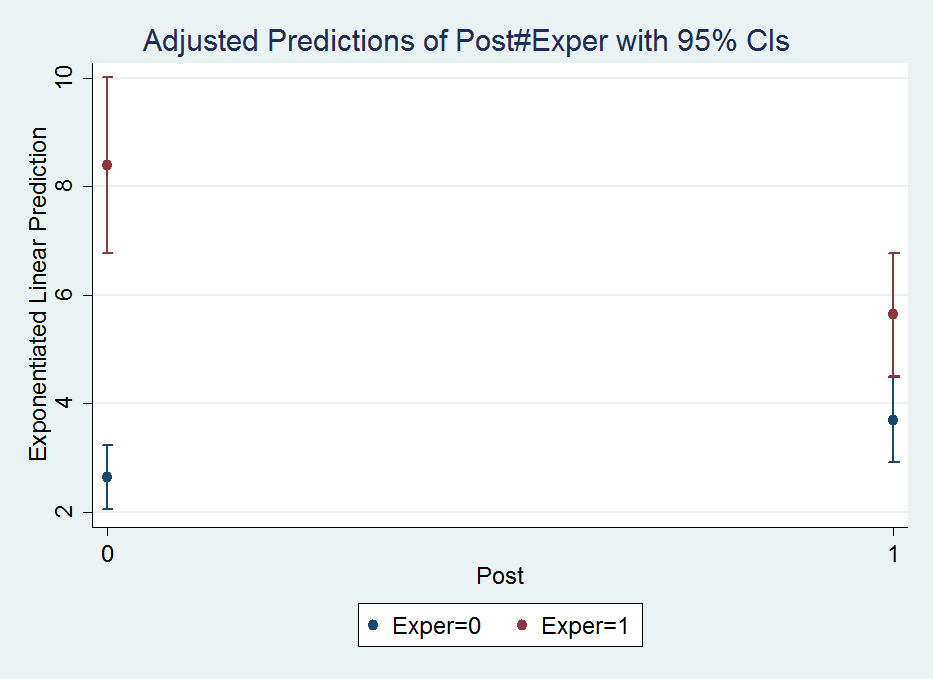The option recast(scatter) draws the plot so there are no lines connecting the different points. Note I prefer to draw these error bar like plots without the end cross hairs, which can be done like marginsplot, recast(scatter) ciopts(recast(rspike)), but this causes a problem when the error bars overlap.

So I initially interpreted this simply as the experimental series went down by around 2 crimes, and the control series went up by around 1 crime. A colleague though pointed out the whole point of having control series though is to say what would have happend if the intervention did not take place (the counterfactual). Because the control series was going up, we would have also expected the experimental series to go up.

The coefficients in this model though don’t directly answer that question. To estimate that relative decrease is somewhat convoluted in this parameterization, but you can use the test and lincom post-estimation commands to do it. Test basically does linear model restrictions for one or multiple variables. This can be useful to test if multiple coefficients are equal to one another, or joint testing to see if one coefficient is equal to another, or to test if a coefficient is different from a non-zero value.

So to test the relative decrease in this DiD setup ends up being (which I am too lazy to explain):

test 1.Exper#1.Post = (1.Exper#0.Post + 0.Exper#1.Post)

Note you can also do a joint test for all dummy variables with testparm:

testparm i.Month

which spits out:

( 1)  2.Month = 0
( 2)  3.Month = 0
( 3)  4.Month = 0
( 4)  5.Month = 0
( 5)  6.Month = 0
( 6)  7.Month = 0
( 7)  8.Month = 0
( 8)  9.Month = 0
( 9)  10.Month = 0
(10)  11.Month = 0
(11)  12.Month = 0

chi2( 11) =   61.58
Prob > chi2 =    0.0000

The idea behind this is that it often does not make sense to test the significance of only one level of a dummy variable – you want to jointly test whether the whole set of dummy variables is statistically significant. Most of the time I do this using F-tests for model restrictions (see this example in R). But here Stata does a chi-square test. (I imagine they will result in the same inferences in most circumstances.)

test just gives inferential statistics though, I wanted an actual estimate of the relative decrease. To do this you can use lincom. So working with my same set of variables I get:

lincom 1.Exper#1.Post - 0.Exper#1.Post - 1.Exper#0.Post

Which produces in the output:

( 1)  - 0b.Exper#1.Post - 1.Exper#0b.Post + 1.Exper#1.Post = 0

------------------------------------------------------------------------------
Y |      Coef.   Std. Err.      z    P>|z|     [95% Conf. Interval]
-------------+----------------------------------------------------------------
(1) |  -.7367337   .1080418    -6.82   0.000    -.9484917   -.5249757
------------------------------------------------------------------------------

I avoid explaining why this particular set of coefficient contrasts produces the relative decrease of interest because there is an easier way to specify the DiD model to get this coefficient directly (see the Wikipedia page linked earlier for an explanation):

*An easier way to estimate the model
xtgee Y i.Exper i.Post Exper#Post i.Month, family(poisson) corr(ar1)

Which you can look at the output and see that the interaction term now recreates the same -.7367337 (.1080418) estimate as the prior lincom command:

Iteration 1: tolerance = .00065297
Iteration 2: tolerance = 1.375e-06
Iteration 3: tolerance = 2.682e-09

GEE population-averaged model                   Number of obs      =       264
Group and time vars:             Exper Ord      Number of groups   =         2
Link:                                  log      Obs per group: min =       132
Family:                            Poisson                     avg =     132.0
Correlation:                         AR(1)                     max =       132
Wald chi2(14)      =    334.52
Scale parameter:                         1      Prob > chi2        =    0.0000

------------------------------------------------------------------------------
Y |      Coef.   Std. Err.      z    P>|z|     [95% Conf. Interval]
-------------+----------------------------------------------------------------
1.Exper |   1.157615   .0803121    14.41   0.000     1.000206    1.315023
1.Post |    .339348   .0898574     3.78   0.000     .1632307    .5154653
|
Exper#Post |
1 1  |  -.7367337   .1080418    -6.82   0.000    -.9484917   -.5249757
|
Month |
2  |    .214908   .1243037     1.73   0.084    -.0287228    .4585388
3  |   .2149183   .1264076     1.70   0.089    -.0328361    .4626726
4  |   .3119988   .1243131     2.51   0.012     .0683497    .5556479
5  |   .4416236   .1210554     3.65   0.000     .2043594    .6788877
6  |    .556197   .1184427     4.70   0.000     .3240536    .7883404
7  |   .4978252   .1197435     4.16   0.000     .2631322    .7325182
8  |   .2581524   .1257715     2.05   0.040     .0116447      .50466
9  |    .222813   .1267606     1.76   0.079    -.0256333    .4712592
10  |    .430002    .121332     3.54   0.000     .1921956    .6678084
11  |   .0923395   .1305857     0.71   0.479    -.1636037    .3482828
12  |  -.1479884   .1367331    -1.08   0.279    -.4159803    .1200035
|
_cons |   .9699886   .1140566     8.50   0.000     .7464418    1.193536
------------------------------------------------------------------------------

But we can do some more here, and figure out the hypothetical experimental mean if the experimental series would have followed the same trend as the control series. Here I use nlcom and exponentiate the results to get back on the original count scale:

nlcom exp(_b[1.Exper] + _b[1.Post]  + _b[_cons])

Which gives an estimate of:

_nl_1:  exp(_b[1.Exper] + _b[1.Post]  + _b[_cons])

------------------------------------------------------------------------------
Y |      Coef.   Std. Err.      z    P>|z|     [95% Conf. Interval]
-------------+----------------------------------------------------------------
_nl_1 |   11.78646   1.583091     7.45   0.000     8.683656    14.88926
------------------------------------------------------------------------------

So in our couterfactual world, the intervention decreased crimes from around 12 to 6 per month instead of 8 to 6 in my original interpretation, a larger effect because of the control series. We can show how nlcom reproduces the output of margins by reproducing the experimental series mean at the baseline, over 8 crimes per month.

*This creates the observed estimates, (at January) - if you want for another month need to add to above
margins Post#Exper, at( (base) Month )
*to show it recreates margins of pre period
nlcom exp(_b[1.Exper] + _b[_cons])

Which produces the output:

. margins Post#Exper, at( (base) Month )

Adjusted predictions                              Number of obs   =        264
Model VCE    : Conventional

Expression   : Exponentiated linear prediction, predict()
at           : Month           =           1

------------------------------------------------------------------------------
|            Delta-method
|     Margin   Std. Err.      z    P>|z|     [95% Conf. Interval]
-------------+----------------------------------------------------------------
Post#Exper |
0 0  |   2.637914   .3008716     8.77   0.000     2.048217    3.227612
0 1  |   8.394722   .8243735    10.18   0.000      6.77898    10.01046
1 0  |   3.703716   .3988067     9.29   0.000     2.922069    4.485363
1 1  |   5.641881   .5783881     9.75   0.000     4.508261    6.775501
------------------------------------------------------------------------------

. *to show it recreates margins of pre period
. nlcom exp(_b[1.Exper] + _b[_cons])

_nl_1:  exp(_b[1.Exper] + _b[_cons])

------------------------------------------------------------------------------
Y |      Coef.   Std. Err.      z    P>|z|     [95% Conf. Interval]
-------------+----------------------------------------------------------------
_nl_1 |   8.394722   .8243735    10.18   0.000      6.77898    10.01046
------------------------------------------------------------------------------

Poisson models are no big deal

Much of my experience suggests that although Poisson models are standard fare for predicting low crime counts in our field, they almost never make a difference when evaluating the marginal effects like I have above. So here we can reproduce the same GEE model, but instead of Poisson have it be a linear model. Here I use the quietly command to suppress the output of the model. Comparing coefficients directly between the two models does not make sense, but comparing the predictions is fine.

*Compare Poisson to a linear model
quietly xtgee Y Exper#Post i.Month, family(gaussian) corr(ar1)
margins Post#Exp, at( (base) Month )

And this subsequently produces:

Adjusted predictions                              Number of obs   =        264
Model VCE    : Conventional

Expression   : Linear prediction, predict()
at           : Month           =           1

------------------------------------------------------------------------------
|            Delta-method
|     Margin   Std. Err.      z    P>|z|     [95% Conf. Interval]
-------------+----------------------------------------------------------------
Post#Exper |
0 0  |   1.864656   .6951654     2.68   0.007     .5021569    3.227155
0 1  |   9.404887   .6951654    13.53   0.000     8.042388    10.76739
1 0  |   3.233312   .6992693     4.62   0.000     1.862769    4.603854
1 1  |   5.810809   .6992693     8.31   0.000     4.440266    7.181351
------------------------------------------------------------------------------

The predictions are very similar between the two models. I simply state this here because I think the parallel trends assumption for the DiD model makes more sense on a linear scale than it does for the exponential scale. Pretty much wherever I look, the effects of explanatory variables appear pretty linear to me when predicting crime counts, so I think linear models make a lot of sense, even if you are predicting low count data, despite current conventions in the field.

An additional Example using marginsplot and areas

Long post – but stick with me! The last thing I wanted to go over was how to get area’s instead of error bars for marginsplot. The pdf help online has some examples – so this is pretty much just a restatement of the help. (Stata’s help in the online PDFs are excellent – code and math and figures and references and intelligible discussion all in one.) Like Andrew Gelman, I think the discrete bars are misleading when the sample locations are just arbitrary locations along a continuous function. I also think the areas look nicer, especially when the error bars overlap.

So using the same example from Dan Kahan, we first use drop _all to get rid of the prior panel data, then use import to load up the csv file.

*This clears the prior data.
drop _all

*grab the csv data
import delimited gelman_cup_graphic_reporting_challenge_data

Now we can estimate our model and our margins:

*estimate a similar logit model
logit agw c.left_right##c.religiosity
*graph areas using margins - pretended -1 and 1 are the high/low religious cut-offs
quietly margins, at(left_right=(-1.6(0.1)1.6) religiosity=(-1 1))

quietly is definately useful here for the margins command, we don’t want to pour through the whole text table, since it is only intended for a chart. And now to make our area chart at the sample points:

marginsplot, xlabel(-1.6(0.4)1.6) recast(line) recastci(rarea) ciopts(color(*.8))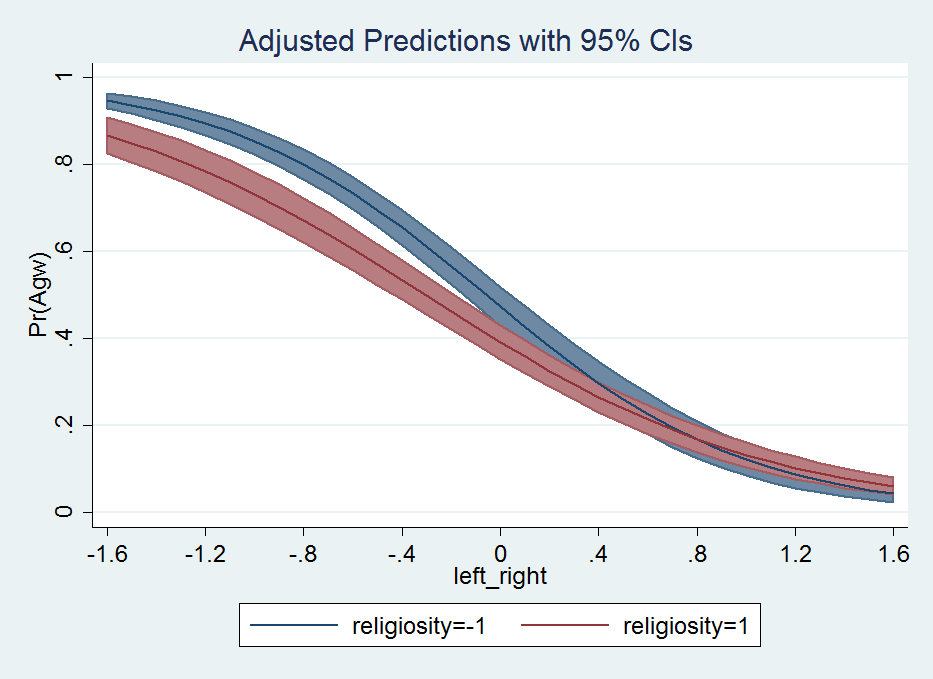That is much better looking that the default with the ugly cross-hair error bars overlapping. Stata draws the lines unfortunately in the wrong order (see the blue line over the red area), and if I figure out an easy way to fix that I will update the post in the future. I need to learn the options for Stata charts to a greater extent before I give any more advice about Stata charts though.

To wrap up. I typically have in my Stata do file:

**************.
*Finish the script.
drop _all
exit, clear
**************.

This drops the dataset (as mentioned before), and exit, clear then basically closes out of Stata.